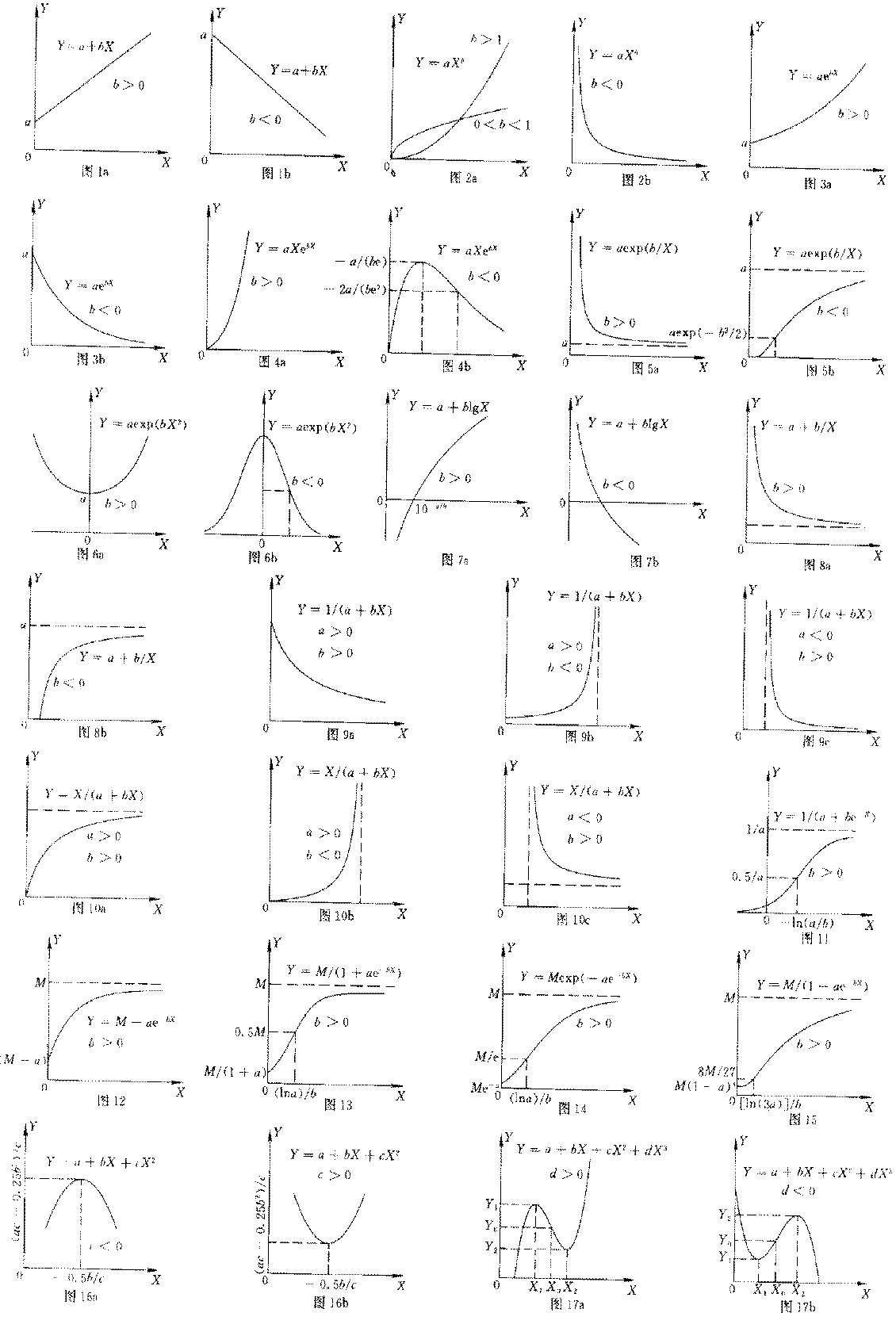----1.曲线回归的可行性判断

2.回归模型的特点

2．1 Y=a＋bX

2．2
Y=aXb

a＞0， 0＜b＜l时，Y 单调递增且下凹，曲线过原点；当a＞0，b＞l时，y单调递增且上凹，曲线过原A，（图2a）；当a＞0， b＜0时，YX 增大单调递减上凹，以X 轴和Y 轴为渐近线（图2b）。2．3 Y=aebX

2．4 Y=aXebX

a＞0， b＞0时，YX 增大而急速上升，曲线通过原点且上凹（图4a）。当a＞0， b＜0时，在X 的区间（0，-1/b）上，Y 单调递增，曲线通过原点；在X=-1／b时，Ymax＝-a /(be）；在X 的区间（-1/b，+∞）上，Y 单调递减，其中，在区间（0，2/b）上，曲线下凹；在区间（-2/b，+∞）上，曲线上凹，向X 轴渐近；拐点坐标为X=-2/b，Y=-2a /(be2（图4b）。

2．5 Y＝aexp(b/X)

2．6  Y＝aexp(bX2)

2. 7 Y＝a＋blgX

b＞0时，YX 增大单调递增（图7a）；当b＜0时，Y单调递减。曲线与X轴的交点坐标为（10-a/b，0）（图7b）。

2．8  Y＝a+b/X

a＞0， b＞0时，曲线有渐近线X＝0Y＝aYX 增大单调递减，且曲线上凹（图8a）；当a＞0，b＜0时，Y单调递增，且曲线下凹，曲线与X轴的交点坐标为（-b/a，0）（图8b）。

2．9 Y＝1/(a＋bX）

2．10 Y＝X/(a＋bX)

a＞0， b＞0时，曲线通过原点，Y  随X   增大单调递增，向Y＝1/b 渐近，曲线下凹（图10a）；当a＞0，b＜0时，曲线通过原点，Y   随X  增大单调递增且向X＝-a／b 渐近，曲线上凹（图10b）；当a＜0，b＞0时，在X 的区间（-a/b，+∞）上Y 取正值，且单调递减，曲线上凹，具有渐近线X=-a／by＝1/b（图10c）。

2．11 Y＝1/(a＋be-X)

a，b均大于零时，有水平渐近线Y＝O和Y＝1／a；拐点坐标为X=-ln（a／b），y＝0.5／a（图11）。

2．12 Y=M-ae-bX

2．13 Y＝M/(l＋ae-bX)

2．14 Y＝Mexp(-ae-bX)

2．15 Y=M(1-ae-bX)3

2．16 Y=a＋bX＋cX2

2.17 Y=a＋bX＋cX2dX3

X0=-c/(3d)代入Y0a＋bX＋cX2dX3 可得拐点坐标（X0Y0）。

3.曲线方程的拟合方法

3．1 一元直线方程

aN＋b∑X＝∑y
a∑x+b∑x2＝∑XY

3．2 可化为一元直线模型的曲线方程

 数学模型 转化方法 转化后形式 Y=aXb U=lgY,V=lgX,A=lga U=A+bV Y=aebx U=lnY,A=lna U=A+bV Y=aXebx U=ln(Y/X),A=lna U=A+bV Y=aexp(b/X) U=lnY,V=1/X,A=lna U=A+bV Y=aexp(bX2) U=lnY,V=X2,A=lna U=A+bV Y=a+blgX V=lgX Y=A+bV Y=a+b/X V=1/X Y=A+bV Y=1/(a+bX) U=1/Y U=A+bV Y=X/(a+bX) U=1/Y,V=1/X U=A+bV Y=1/(a+be-x) U=1/Y,V=e-X U=A+bV Y=M-ae-bX U=ln(M-Y),V=-X,A=lna U=A+bV Y=M/(1+ae-bX) U=ln(M-Y)/Y,V=-X,A=lna U=A+bV Y=Mexp(-ae-bX) U=ln[-ln(Y/M)],V=-X,A=lna U=A+bV Y=M(1-ae-bX)3 U=ln[1-(Y/M)1/3,V=-X,A=lna U=A+bV

3.3 二次抛物线方程

aN+b∑X+c∑X2=∑y
a∑X+b∑X2+c∑X3=∑XY
a∑X2+b∑X3+c∑X4=∑X2Y

3.4 三次抛物线方程

aN+b∑X+c∑X2+dX3=∑y
a∑X+b∑X2+c∑X3+dX4=∑XY
a∑X2+b∑X3+c∑X4+d∑X5=∑X2Y
a∑X3+b∑X4+c∑X5+d∑X6=∑X3Y

4.曲线方程的显著性判断

1 袁志发，顾天骥，主编。概率基础与数理统计。北京：农业出版社, 1988.221-237.
2 俞渭江，主编。生物统计：附试验设计，北京：农业出版社，1980. 114-130.
3 张文举，徽成盆地黄牛生长曲线方程的研究。甘肃畜牧兽医。1998,28（1）:3-6.
4 郭祖超，编。医用数理统计方法。北京：人民卫生出版社，1963．414-458.
5 符伍儒，主编，数理统计，北京：农业出版社， 1980．223一234.
----